Back to Contents

# Functions upon Functions upon Functions

Often we need to apply a function to every element of a list. For example, doubling each of the numbers in a list of integers. We could do this with a simple recursive function, working over each element of a list: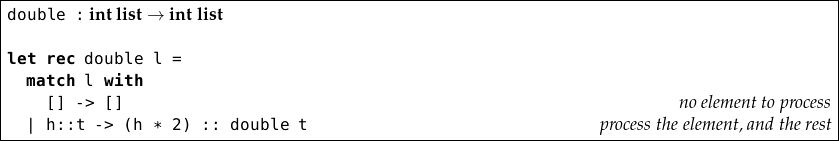For example,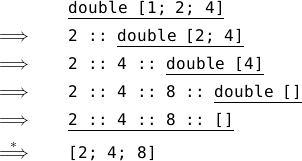The result list does not need to have the same type as the argument list. We can write a function which, given a list of integers, returns the list containing a boolean for each: `true` if the number is even, `false` if it is odd.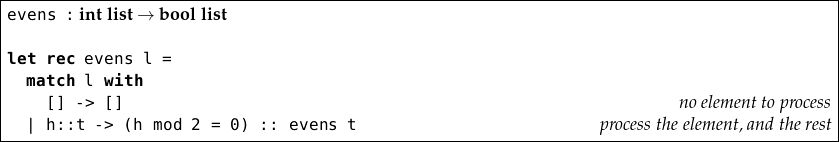For example,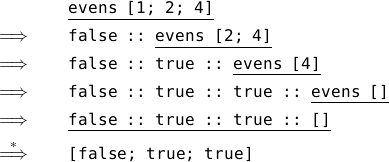It would be tedious to write a similar function each time we wanted to apply a different operation to every element of a list – can we build one which works for any operation? We will use a function as an argument: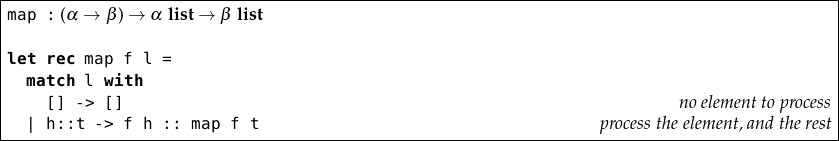The `map` function takes two arguments: a function which processes a single element, and a list. It returns a new list. We will discuss the type in a moment. For example, if we have a function `halve`: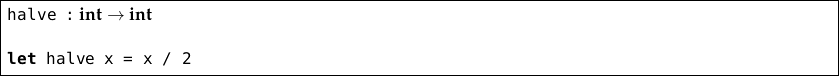We can use `map` like this: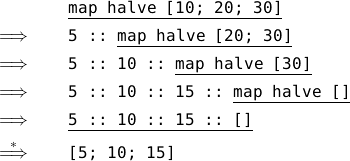Now, let us look at that type: (α β) α list β list. We can annotate the individual parts: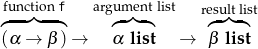We have to put the function `f` in parentheses, otherwise it would look like `map` had four arguments. It can have any type α β. That is to say, it can have any argument and result types, and they do not have to be the same as each other – though they may be. The argument has type α list because each of its elements must be an appropriate argument for `f`. In the same way, the result list has type β list because each of its elements is a result from `f` (in our `halve` example, α and β were both int). We can rewrite our `evens` function to use `map`: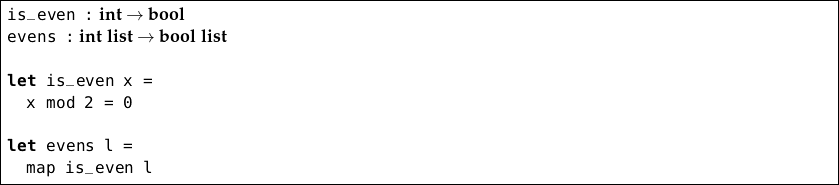In this use of `map`, α was int, β was bool. We can make `evens` still shorter: when we are just using a function once, we can define it directly, without naming it: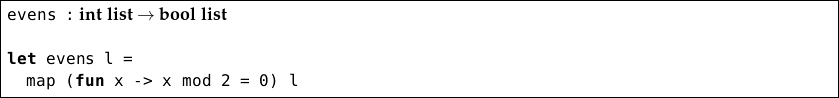This is called an anonymous function. It is defined using `fun`, a named argument, the `->` arrow and the function definition (body) itself. For example, we can write our halving function like this:

`fun`` x -> x / 2`

and, thus, write: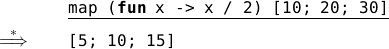We use anonymous functions when a function is only used in one place and is relatively short, to avoid defining it separately.

In the preceding chapter we wrote a sorting function and, in one of the questions, you were asked to change the function to use a different comparison operator so that the function would sort elements into reverse order. Now, we know how to write a version of the `msort` function which uses any comparison function we give it. A comparison function would have type α α bool. That is, it takes two elements of the same type, and returns `true` if the first is “greater” than the second, for some definition of “greater” – or `false` otherwise.

So, let us alter our `merge` and `msort` functions to take an extra argument – the comparison function: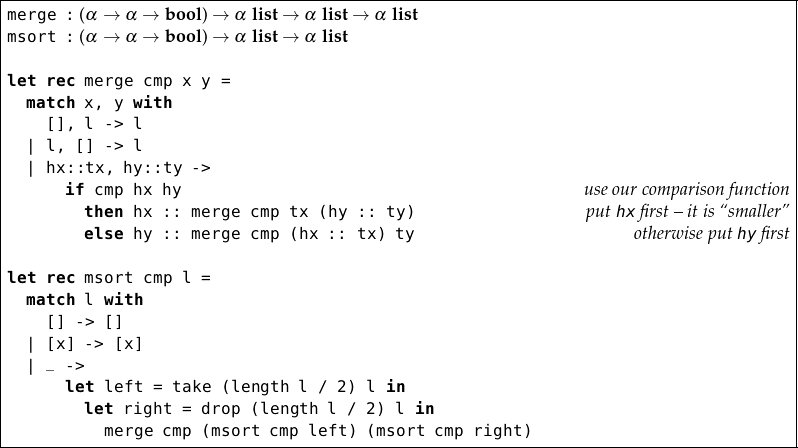Now, if we make our own comparison operator: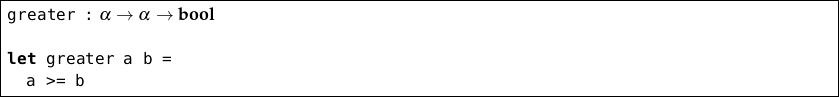we can use it with our new version of the `msort` function: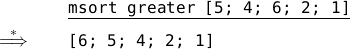In fact, we can ask OCaml to make such a function from an operator such as `<=` or `+` just by enclosing it in parentheses and spaces:

` OCaml`

`# ( <= )`
`- : 'a -> 'a -> bool = <fun>`
`# ( <= ) 4 5`
`- : bool = true`

So, for example: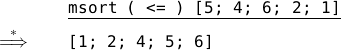and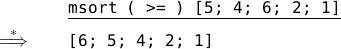The techniques we have seen in this chapter are forms of program reuse, which is fundamental to writing manageable large programs.

## Questions

1. Write a simple recursive function `calm` to replace exclamation marks in a char list with periods. For example `calm [’H’; ’e’; ’l’; ’p’; ’!’; ’ ’; ’F’; ’i’; ’r’; ’e’; ’!’]` should evaluate to `[’H’; ’e’; ’l’; ’p’; ’.’; ’ ’; ’F’; ’i’; ’r’; ’e’; ’.’]`. Now rewrite your function to use `map` instead of recursion. What are the types of your functions?

2. Write a function `clip` which, given an integer, clips it to the range 1…10 so that integers bigger than 10 round down to 10, and those smaller than 1 round up to 1. Write another function `cliplist` which uses this first function together with `map` to apply this clipping to a whole list of integers.

3. Express your function `cliplist` again, this time using an anonymous function instead of `clip`.

4. Write a function `apply` which, given another function, a number of times to apply it, and an initial argument for the function, will return the cumulative effect of repeatedly applying the function. For instance, `apply f 6 4` should return `f (f (f (f (f (f 4))))))`. What is the type of your function?

5. Modify the insertion sort function from the preceding chapter to take a comparison function, in the same way that we modified merge sort in this chapter. What is its type?

6. Write a function `filter` which takes a function of type α bool and an α list and returns a list of just those elements of the argument list for which the given function returns `true`.

7. Write the function `for_all` which, given a function of type α bool and an argument list of type α list evaluates to `true` if and only if the function returns `true` for every element of the list. Give examples of its use.

8. Write a function `mapl` which maps a function of type α β over a list of type α list list to produce a list of type β list list.